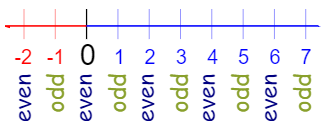# URI / BEE CROWD 1074 - Even or Odd Solution in C,C++,Python | URI - BEECROWD - BEE 1074 Solution in C,C++,Python

beecrowd | 1074

# Adapted by Neilor Tonin, URI  Brazil

Timelimit: 1

Read an integer value N. After, read these N values and print a message for each value saying if this value is oddevenpositive or negative. In case of zero (0), although the correct description would be "EVEN NULL", because by definition zero is even, your program must print only "NULL", without quotes.

## Input

The first line of input is an integer (< 10000), that indicates the total number of test cases. Each case is a integer number (-107 < X <107)..

## Output

For each test case, print a corresponding message, according to the below example. All messages must be printed in uppercase letters and always will have one space between two words in the same line.

 Input Sample Output Sample 4-503-4 ODD NEGATIVENULLODD POSITIVEEVEN NEGATIVE

### Demonstration:

Even numbers are those numbers that can be divided into two equal groups or pairs and are exactly divisible by 2.
Any integer that cannot be divided exactly by 2 is an odd number.A number is positive if it is greater than zero.  A number is negative if it is less than zero.
Here we apply a range-based for loop which provides all the integers available in the input interval.
After this, checking the condition for even,odd,positive and negative numbers are applied and increment these variable.
Finally, print the result.
N.B: Don't copy paste the code as same. Just try to understand it and try yourself.

## URI / BEECROWD Online Judge 1074 Solve in C :

```#include <stdio.h>
int main()
{
int N,X,a;
scanf ("%d", &N);
for(a=1;a<=N;a++)
{
scanf ("%d",&X);
if(X==0)
printf("NULL\n");
else if(X<=0&&X%2==0)
printf ("EVEN NEGATIVE\n");
else if(X<=0&&X%2==-1)
printf ("ODD NEGATIVE\n");
else if(X>=0&&X%2==0)
printf ("EVEN POSITIVE\n");
else if(X>=0&&X%2==1)
printf ("ODD POSITIVE\n");
}
return 0;
}```